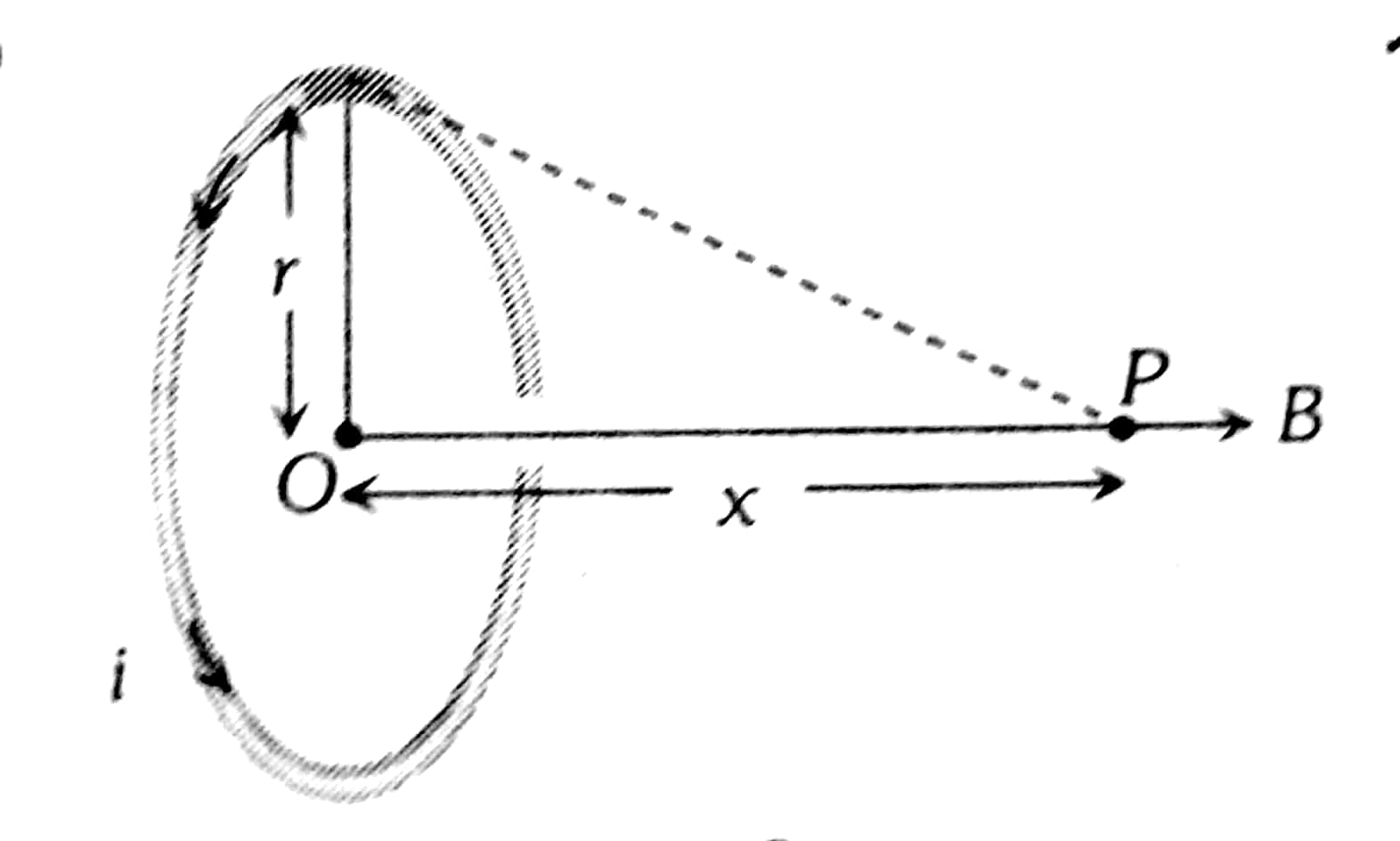# The magnetic field due to a current carrying circular loop of radius 3 cm at a point on the axis at a distance of 4 cm from the centre is . What will be its value at the centre of the loop ? Option 1) Option 2) Option 3) Option 4)

V Vakul

As we discussed in

Magnetic Field at the axis due to circular current carrying wire -

- wherein

N is numbers of turn in coil

Magnetic Field due to Circular Current at the centre -

If a coil of radius r is carrying current i then magnetic feild at a distnace X from its centre is:

- whereinOption 1)

Correct option

Option 2)

Incorrect option

Option 3)

Incorrect option

Option 4)

Incorrect option

Exams
Articles
Questions